# Summer Fun Multiplication Puzzles

Looking for a fun way to practice multiplication during summer? Check out these FREE multiplication puzzles for some summer fun. These FREE summer fun multiplication puzzles are easy to prep and learners can learn and have fun at the same time. Simple download the summer fun multiplication puzzles printables pdf file and great ready make learning multiplication for 2nd grade, 3rd grade, and 4th grade students FUN!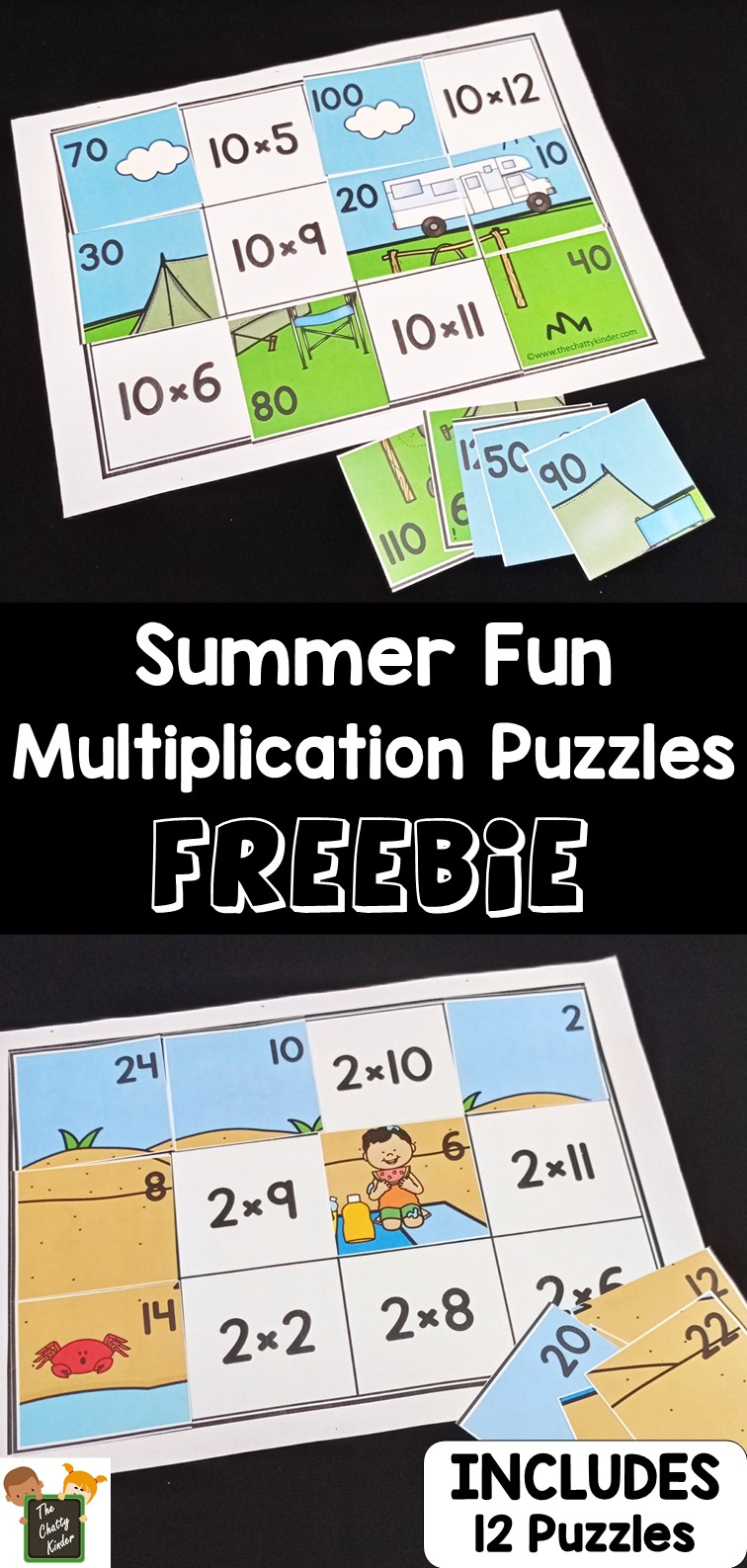## Multiplication Puzzles – Free Printable

Scroll to the bottom of this post and enter in your details in the YELLOW box at the end of this post. You should receive an email with the printable. Do check your junk or spam email, as it might have ended up there as well. If you have any issues please contact me at thechattykinder@gmail.com

Once kids are familiar with their multiplication facts they are ready to use this easy way to practice their multiplication facts. Mastering multiplication facts requires a lot of practice in order to make the concepts click and for them to retain the multiplication facts. And although multiplication worksheets are a easy way to do that, they are not as engaging for kids. Use these multiplication puzzles as a fun, free multiplication printable to make math fun for grade 2, grade 3, and grade 4 students. Whether you are a parent, teacher, or homeschooler – you will love this hands-on multiplication activity.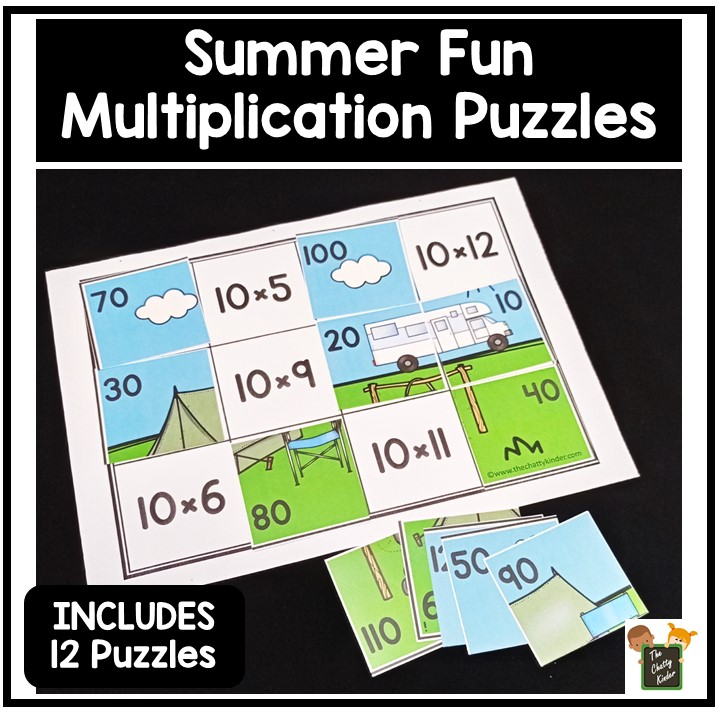## Teaching Multiplication

I love teaching maths in general because it is a subject that can be taught using many visual and hands on aids. These aids can be using manipulatives such as base 10 blocks or hands on math stations and centers. In this post, I will be sharing an interactive hands on printable which can be used to teach multiplication and is an ideal addition into math centers. Hopefully, this printable will help to make teaching multiplication at your home or classroom more enjoyable.

Trucker has recently started learning multiplication and he is quite familiar with a couple of the multiplication tables. I wanted to create a fun printable that would incorporate the multiplication facts into a fun learning activity.

When teaching multiplication it is important that children get the concept before just memorizing the multiplication facts. This is so that they are able to apply these concepts into their daily lives (for e.g. in counting money). So if you are looking for some fun ways to teach multiplication using repeated addition and arrays check out the printables at the end of this post. If you would like to read more about how to teach multiplication check out this post over here. I taught Trucker the concepts of multiplication using the fact booklets and the multiplication puzzles so be sure to check these out.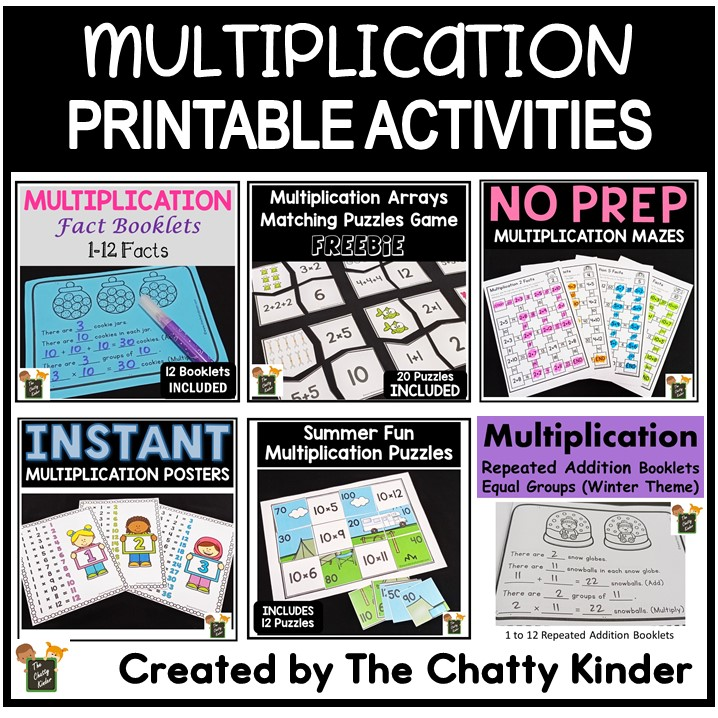## Multiplication Puzzles – A Fun and Easy Way to Practice Multiplication

I absolutely LOVE puzzles! And Trucker has started enjoying puzzles a lot more, so it is the perfect opportunity for me to combine maths and puzzles together to create some fun learning activities. These multiplication puzzles are quite simply my favourite puzzles that I’ve created so far.

In these multiplication puzzles learners will have to solve the multiplication equation on the grid. Then they will have to pick up the puzzle piece that contains that number of the solved multiplication fact and place it on that square. For e.g. if the multiplication in the square is 2×2, then the learner will have to know that the answer to this fact is 4, and thus will have to pick up the puzzle piece which has the number 4 on it and place it on to the multiplication equation of 2×2.

This is an ideal hands-on practice to firm up the multiplication facts that the learner is already familiar with. The printable contains puzzles all the way from 1 to 12 Facts for a total of 12 puzzles.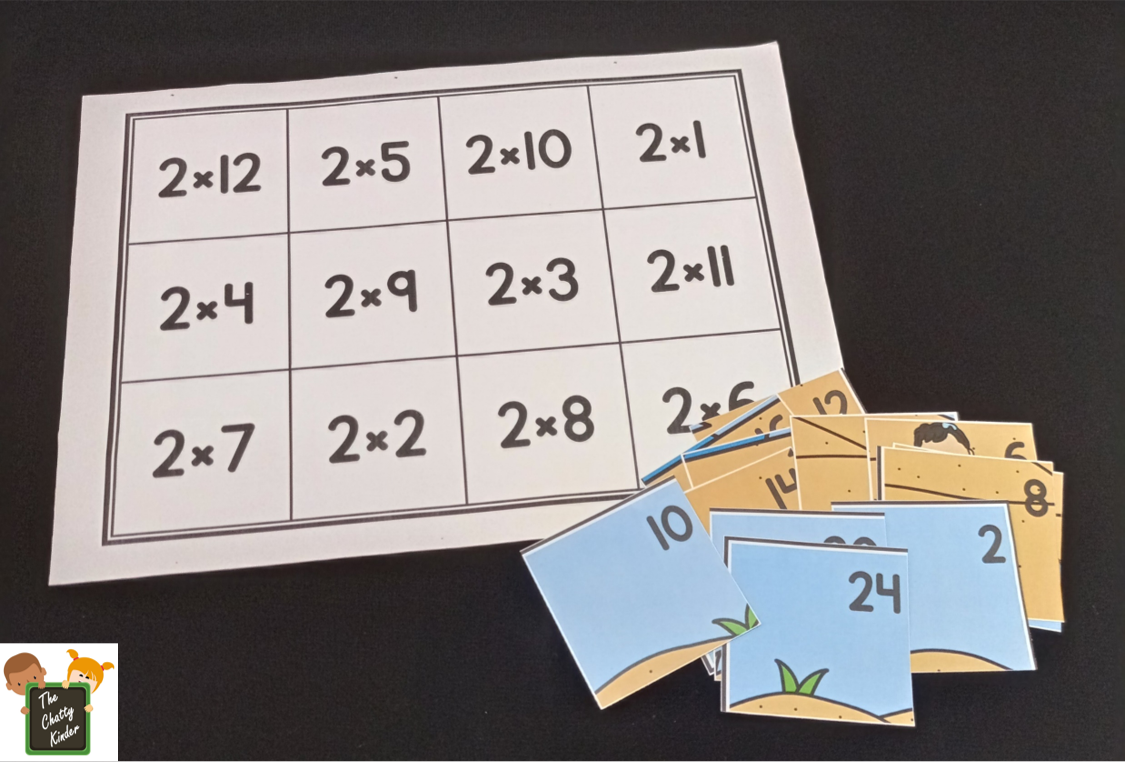## Summer Fun Puzzles

These puzzles are summer themed and are perfect for practicing multiplication concepts for your second graders, or to review multiplication concepts for your third or fourth graders.

## Differentiation:

The printable contains a total of 12 puzzles, one for each of the puzzle facts. This activity is extremely simple to differentiate. Simply choose the puzzle that you would like your learner to practice on and give it to them. This way if one learner needs to work on their 2 Facts while another needs to work on their 5 Facts, both learners can practice on their facts.

## Materials Required:

### To Use:

• Print the file and laminate (this is recommended, if you are planning to re-use the puzzles)
• Cut apart the puzzle page to separate it into the square puzzle pieces.
• Keep the grid containing the multiplication equations as is.

## Presentation:

• First, decide which multiplication fact you’d like to review with your learners. Each puzzle focuses on only one of the multiplication facts, so be sure to pick which one you want.
• Before starting the puzzle, I would recommend you to revise the multiplication facts so students are familiar with their facts before they start on the puzzles.
• This activity can be done individually or in pairs. Give the learner the page containing the multiplication facts and the cut apart pieces of the matching puzzle set. The learner will look at the multiplication equation and solve the equation. Then the learner will take the piece of the puzzle that has the matching number and place it on the grid matching the equation.
• For e.g. in the following picture, 3×3 is 9, so the leaner will take the piece of the puzzle that has 9 on it and place it on 3×3. In this way the learner will continue to solve each fact and place the corresponding puzzle piece with the matching number. As each fact gets solved, the puzzle will slowly come together.
• In this activity, students will use both their puzzle solving skills as well as being able to practice their multiplication facts making this a fun and enriching learning activity for multiplication.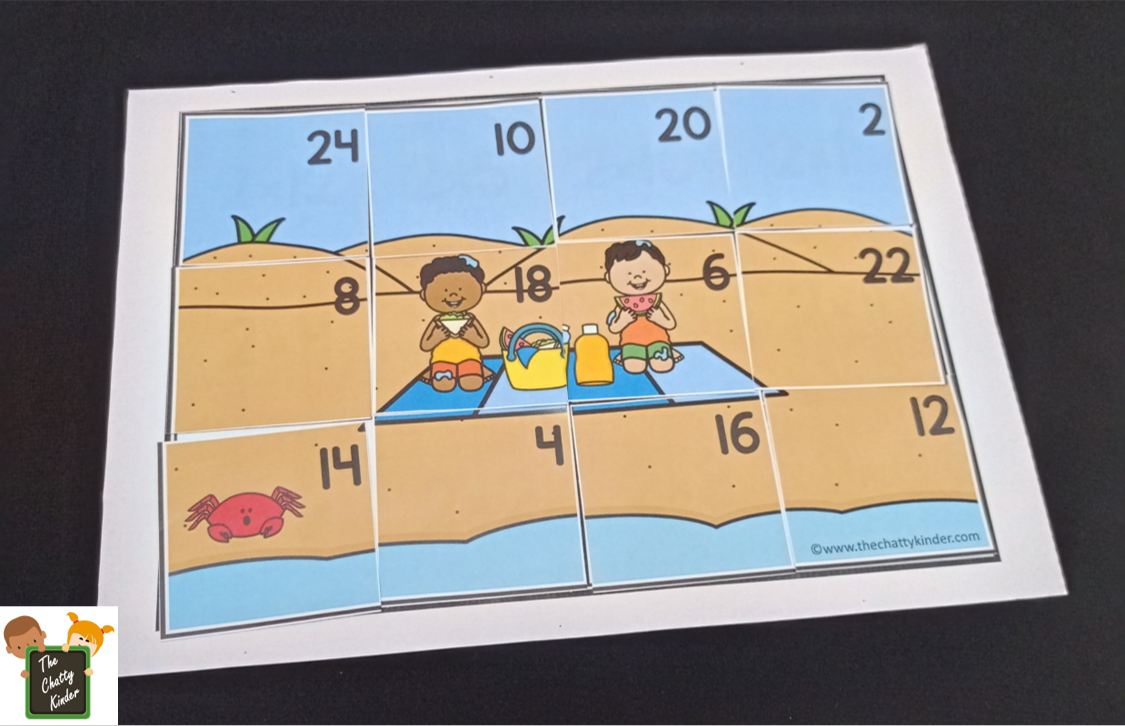This multiplication math activity, when done a few times will help to firm up the maths concepts and make practicing multiplication facts so much easier and fun.

## More Multiplication Printables:

Enter your details into the yellow box below to grab your FREE Summer Fun Multiplication Puzzles Printable! Enjoy!